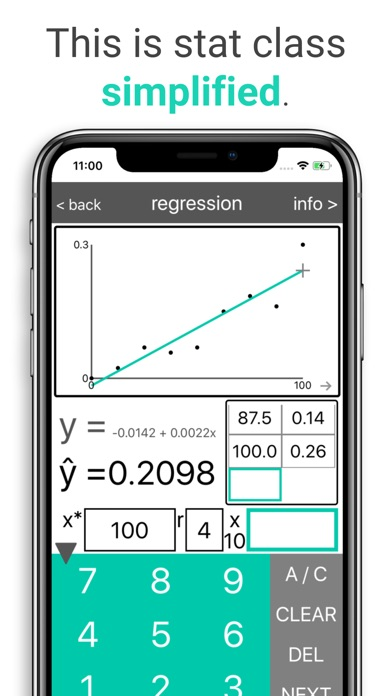# statmagic PRO

## iOS UniversalEducation

Free

Statmagic PRO has helped thousands of students finish their statistics homework faster. It eliminates tedious number-crunching, and helps you avoid common mistakes -- all while making intro stats simpler, clearer, and easier to learn.

Statmagic PRO is the best, easiest-to-use app for learning stats. Why?
• Simplicity. Statmagic uses a simple and familiar calculator layout.
• Complete results. From the standard error to the Z score, to the p-value and "reject Ho?" conclusion, Statmagic calculates and returns every important value. And Statmagic PRO’s 11+ calculators have been tested exhaustively to ensure that they return correct answers, first and foremost.
• Press and hold definitions. Every input field has an on-the-spot definition, so you can quickly remind yourself what each statistical symbol means, and enter the right value for it.
• Error checking. Statmagic automatically checks for typos and anomalous numbers, to help you prevent simple mistakes. For example, if you select a Z test when n is < 30 and the standard deviation is not known, Statmagic will politely suggest a T test instead.
• An exceedingly easy dataset import feature. Just open a spreadsheet in a spreadsheet app, copy the data onto your device's clipboard, and Statmagic can read it. Running a regression is then just a click away! Or, Statmagic will help you select the correct test for your 1-sample, 2-sample, or paired data.

Plus:
• User-specified answer rounding. Statmagic will round its answers to any number of digits that you specify.
• Automatic graphics and plots, so you can visualize what’s going on. For instance, you can finally skip the confusing Z table -- Statmagic automatically graphs the area under the curve, for any Z or T score!
• Extensive, yet easy-to-navigate help material, with straightforward explanations of the logic and formulas of statistics. All honed by the developer’s years of one-on-one stat tutoring experience.
• Integration with Statmagic's online learning videos, which explains the core ideas of a semester-long stat course in less time than 2 lectures (videos are in English only).

With its 11+ optimally-designed calculators, Statmagic covers the most common topics of intro stat classes, each with its own help page and memorable example problems. These are:
• Descriptive stats (mean, quartiles, standard deviation, etc.).
• Probabilities (Z and T scores) on the normal distribution.
• One- and two-sample tests of mean (Z, T, and Welch tests).
• One- and two-sample tests of proportion (allowing both decimal "0.48" and fractional "54/112" type entries for p).
• Confidence intervals (for a mean or a proportion, featuring a full, auto-filling CI formula, so you can see how the CI is constructed).
• Minimum N of a sample (for measuring a true mean or a proportion within a set margin of error).
• Least-squares linear regression (with an automatic scatterplot coloring outliers, and a tool to calculate the predicted y for any chosen x using the regression equation).
• The best-designed Chi-squared calculator out there. (Which, besides furnishing full test results, including the critical Chi-square and p-values, instantly calculates all the expected and Chi-squared values as soon as you enter the observed counts. Handles up to a 7x7 table.)

Learn statistics efficiently, and finish your stats homework faster. Learn, practice, and master stats with Statmagic PRO!

## What's New

• Improved the flow of the first launch.

•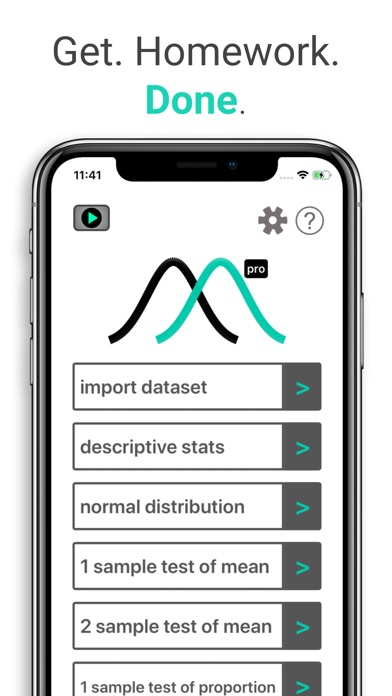•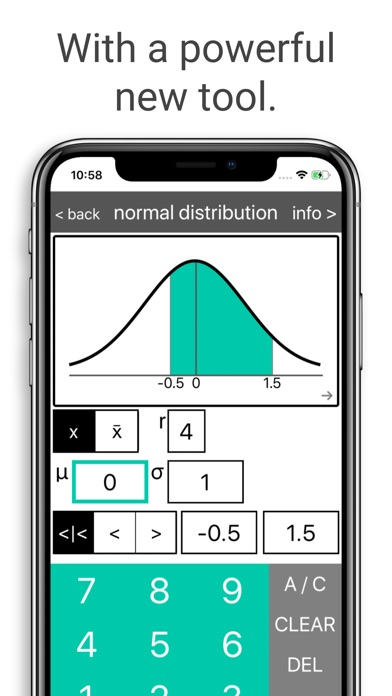•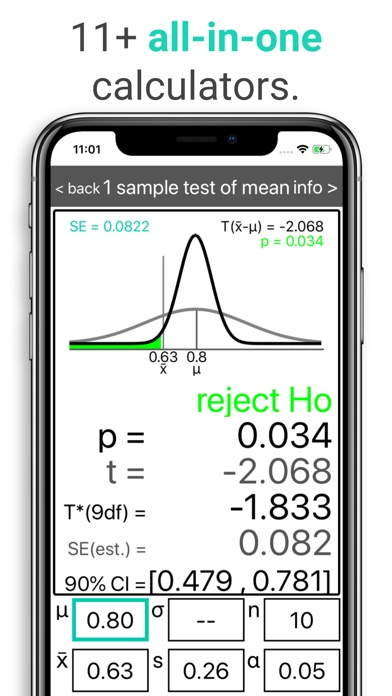•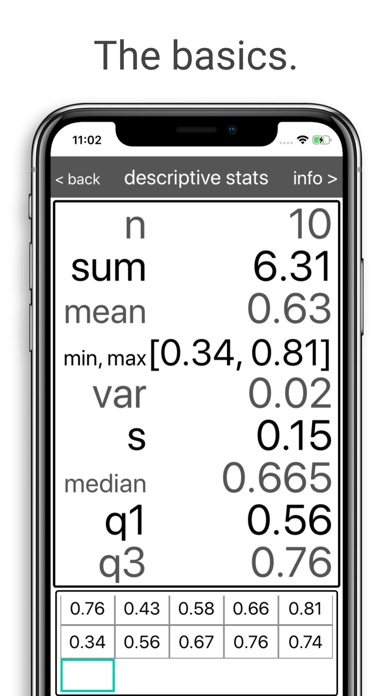•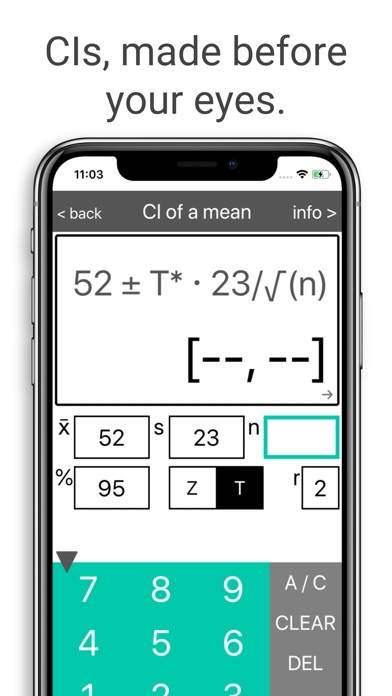•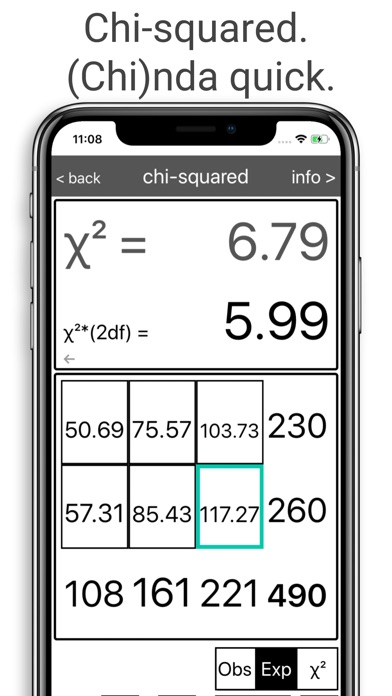•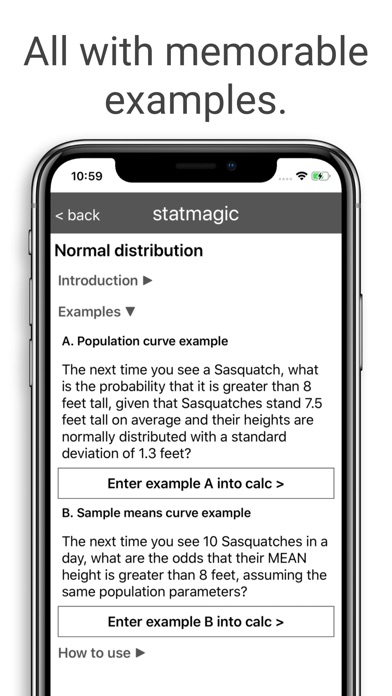•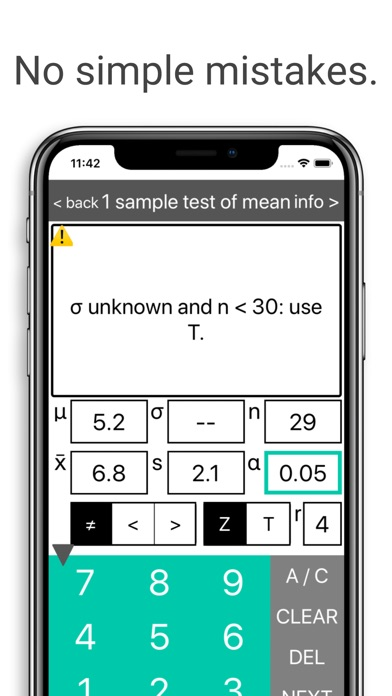•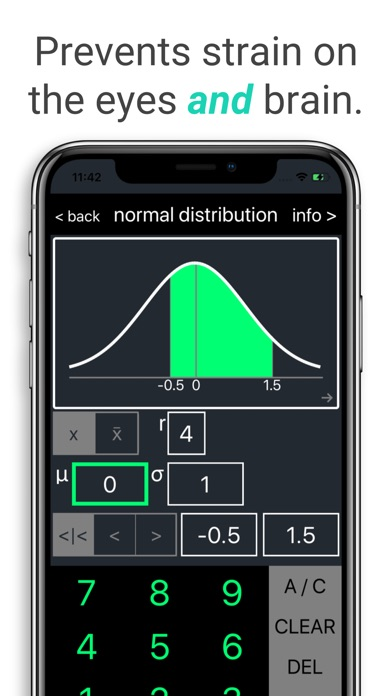•